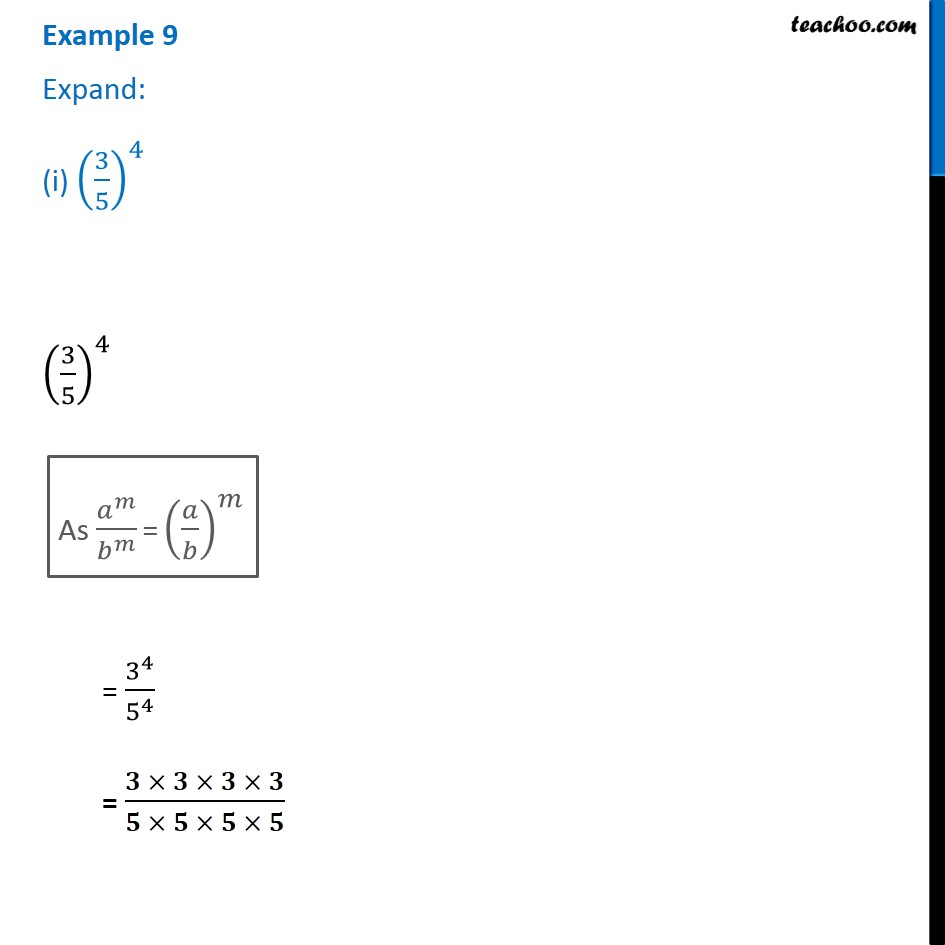1. Chapter 13 Class 7 Exponents and Powers
2. Concept wise
3. Law of exponents

Transcript

Example 9 Expand: (i) (3/5)^4 (3/5)^4 As 𝑎^𝑚/𝑏^𝑚 = (𝑎/𝑏)^𝑚 (3/5)^4 = 3^4/5^4 = (𝟑 × 𝟑 × 𝟑 × 𝟑)/(𝟓 × 𝟓 × 𝟓 × 𝟓)